# 1. Introducing leastcostpath

This vignette describes leastcostpath, a package written for use in the R environment (R Core Team, 2018) and built on classes and functions provided in the R package gdistance (Van Etten, 2017).

leastcostpath provides the functionality to calculate Least Cost Paths (LCPs) using numerous time- and energy-based cost functions that approximate the difficulty of moving across a landscape. Additional cost surfaces can be incorporated into the analysis via create_barrier_cs() or create_feature_cs().

leastcostpath provides the functionality to calculate Stochastic Least Cost Paths (Pinto and Keitt, 2009), and Probabilistic Least Cost Paths (Lewis, 2020).

leastcostpath provides the functionality to calculate movement potential within a landscape through the implementation of From-Everywhere-to-Everywhere (White and Barber, 2012), Cumulative Cost Paths (Verhagen 2013), and Least Cost Path calculation within specified distance bands (Llobera, 2015).

leastcostpath provides the functionality to validate the accuracy of the computed Least Cost Path relative to another path via validate_lcp() (Goodchild and Hunter, 1997) and PDI_validation() (Jan et al. 1999).

# 2. using leastcostpath

## 2.1 Setup

library(rgdal)
library(rgeos)
library(sp)
library(raster)
library(Matrix)
library(gdistance)
library(leastcostpath)
dem <- raster::raster(system.file('external/maungawhau.grd', package = 'gdistance'))
raster::crs(dem) <- sp::CRS("+init=epsg:27200")

dem_extent <- as(raster::extent(dem), 'SpatialPolygons')
raster::crs(dem_extent) <- raster::crs(dem)

raster::plot(dem)
raster::plot(dem_extent, add = T, border = "red")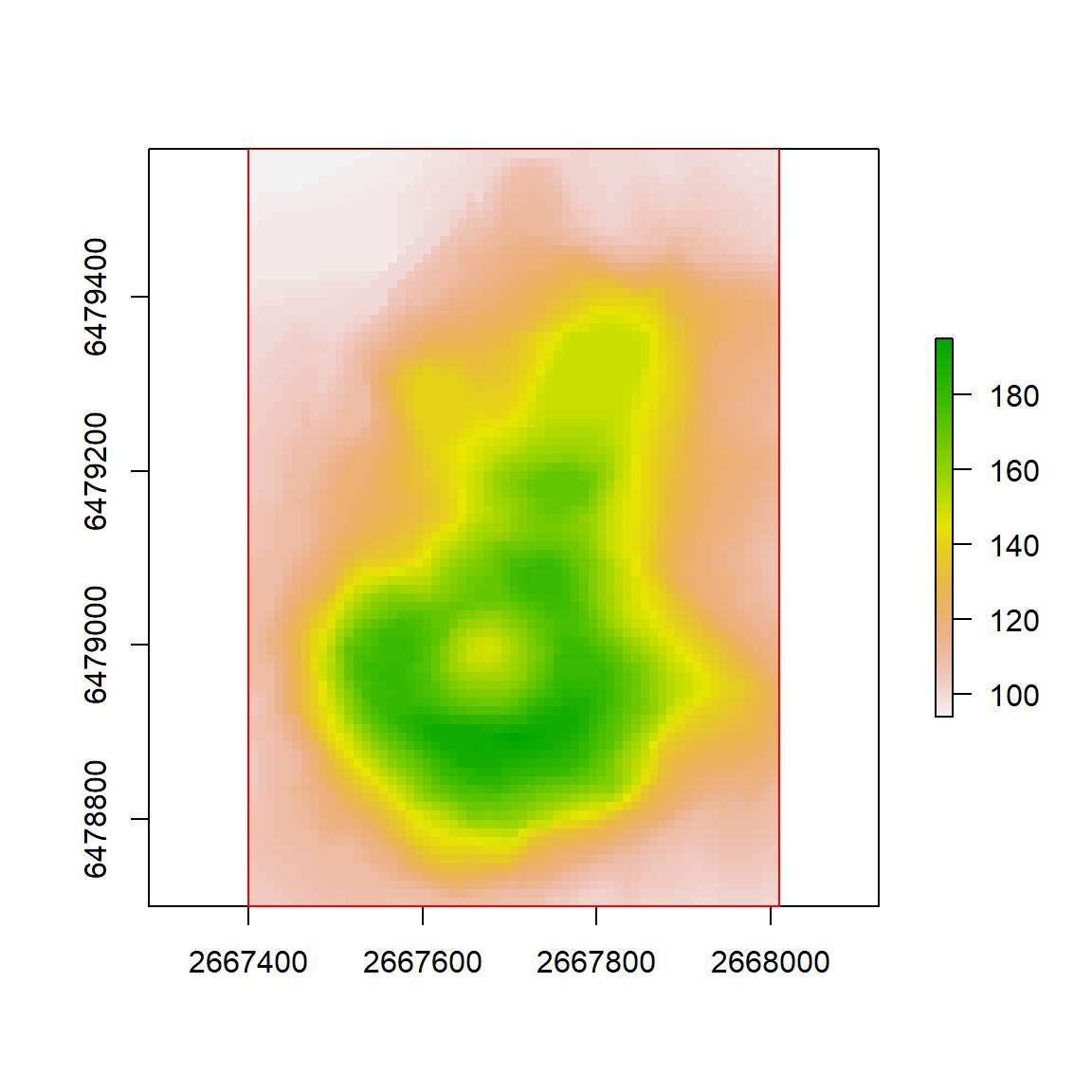## 2.2 Creating Slope-based Cost Surfaces

# cost functions currently implemented within leastcostpath

cfs <- c("tobler", "tobler offpath", "irmischer-clarke male",
"irmischer-clarke offpath male", "irmischer-clarke female",
"irmischer-clarke offpath female","modified tobler",
"wheeled transport", "herzog", "llobera-sluckin", "campbell 2019")

# neighbours can be 4, 8, 16, 32, or 48. 4 used for illustration purposes, but greater number of neighbours = cost surface and LCP approximates reality better.

neigh <- 4

slope_cs <- leastcostpath::create_slope_cs(dem = dem, cost_function = "tobler", neighbours = neigh)

plot(raster(slope_cs), col = grey.colors(100))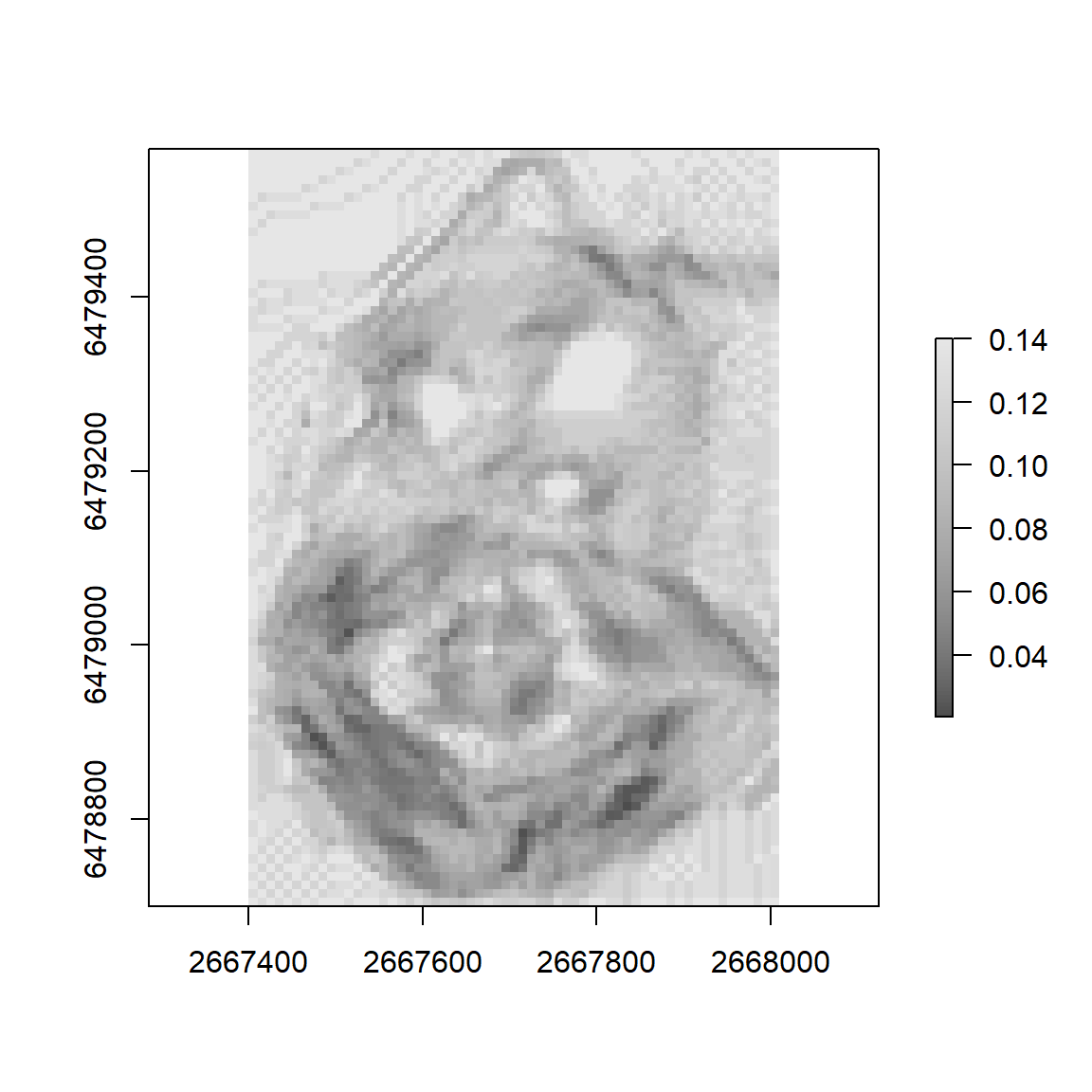## 2.3 Creating and Incorporating Barriers within Cost Surfaces

# create barrier of altitude values above 180

altitude <- dem > 180
# convert value 0 to NA - this ensures that the areas below 180 are not viewed as barriers. That is, if the raster value is NA then create_barrier_cs() will assume that the cell is background and will therefore assign the background argument value
altitude[altitude == 0] <- NA

# the values NOT NA will be assigned the field argument value. If 0 (default) then movement within the area will be completely prohibited
altitude_cs <- leastcostpath::create_barrier_cs(raster = altitude, barrier = altitude, neighbours = neigh, field = 0, background = 1)

# multiplying the two cost surfaces ensures that barriers continue to completely inhibit movement (i.e. slope_cs values * 0 = 0)
slope_altitude_cs <- slope_cs * altitude_cs

plot(raster(slope_altitude_cs), col = grey.colors(100))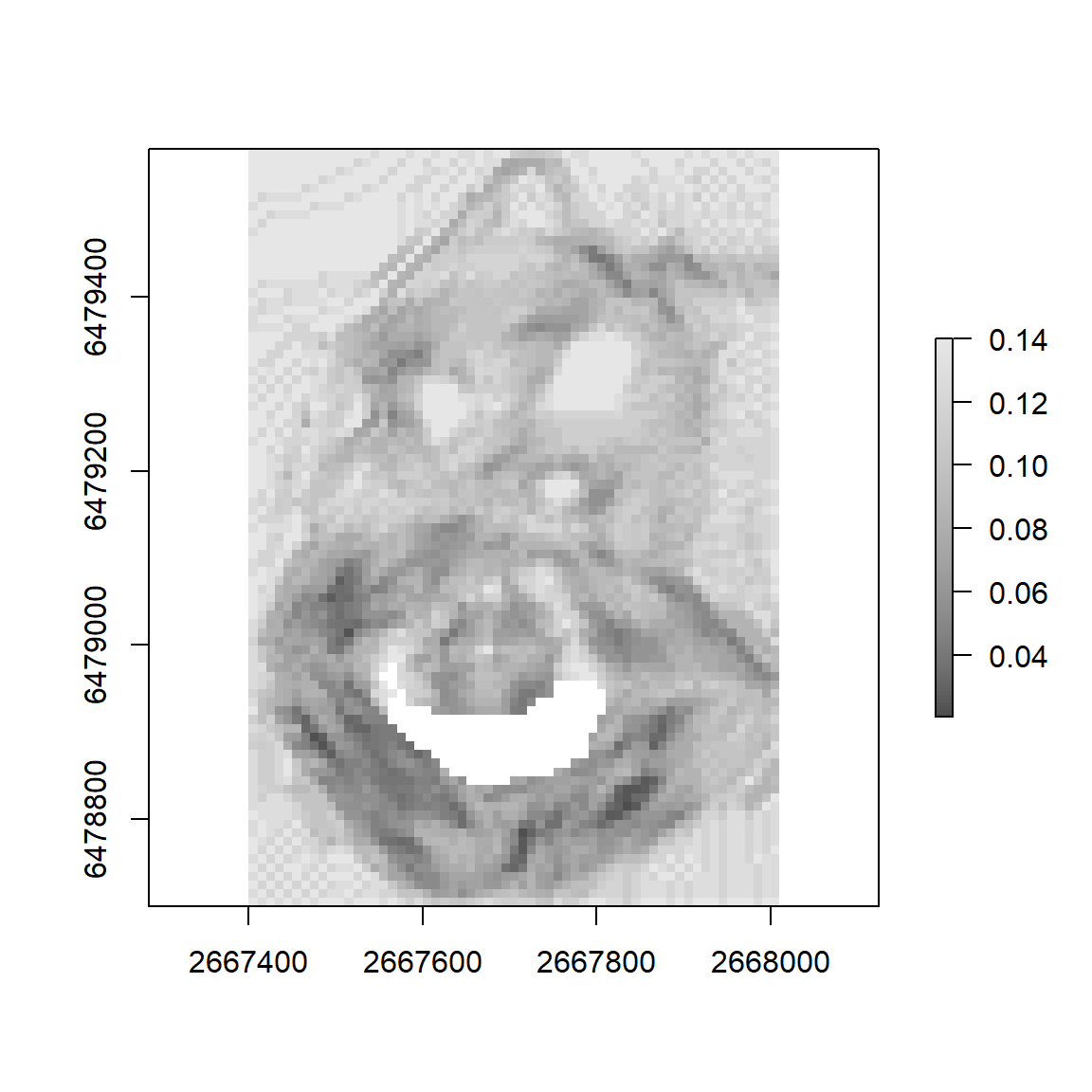## 2.3 Calculating Least Cost Paths

A <- sp::SpatialPoints(cbind(2667930, 6479466))
B <- sp::SpatialPoints(cbind(2667668, 6478818))

# if cost function is anisotropic (e.g. Tobler's Hiking Function) then LCP from A-to-B and B-to-A may be different. To create LCP in both directions set the directional argument to FALSE (default)
lcp <- leastcostpath::create_lcp(cost_surface = slope_altitude_cs, origin = A, destination = B, directional = FALSE)

plot(raster(slope_altitude_cs), col = grey.colors(100))
plot(A, add = T, col = "black")
plot(B, add = T, col = "black")
# LCP from A-to-B
plot(lcp[1,], add = T, col = "red")
# LCP from B-to-A
plot(lcp[2,], add = T, col = "blue")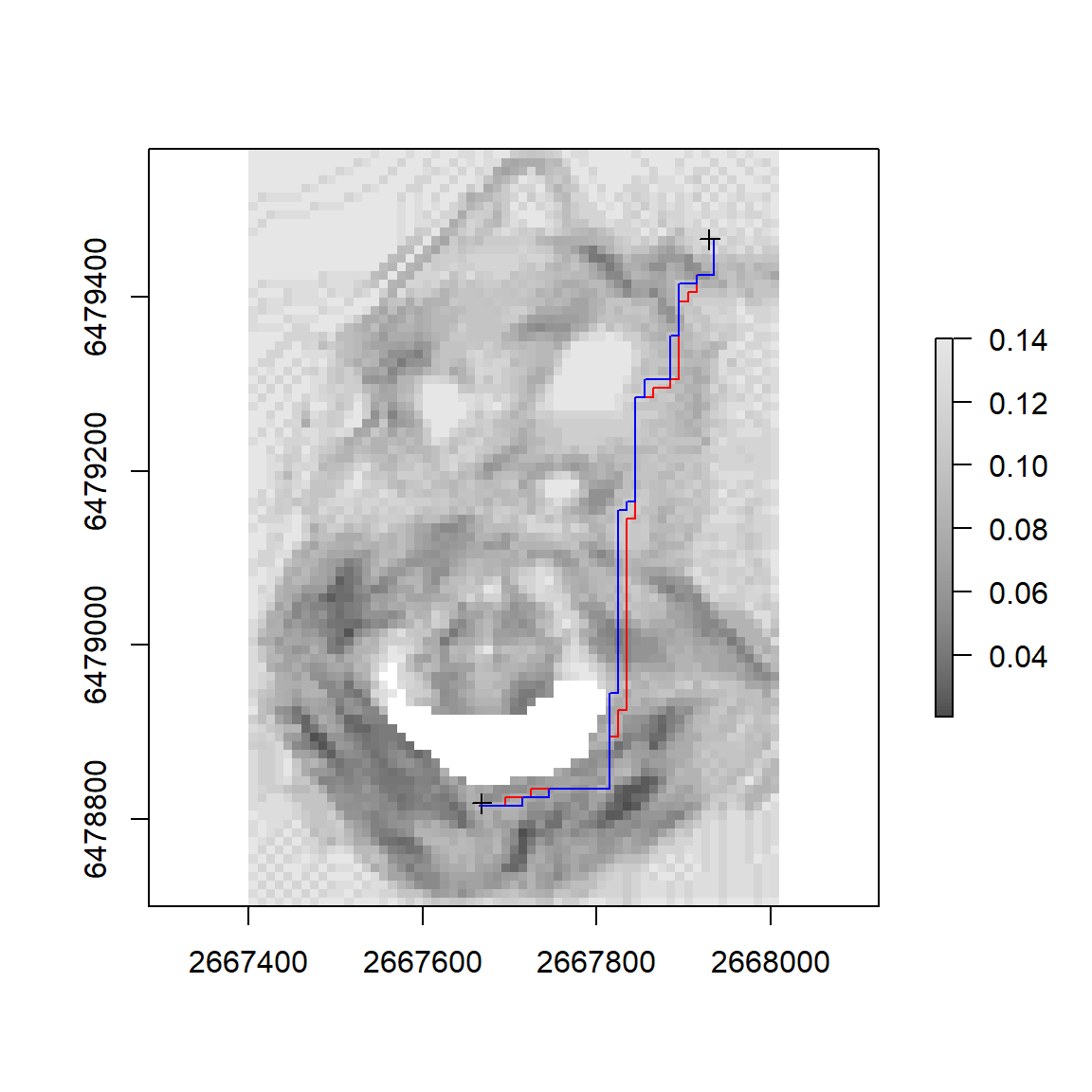## 2.4 Validating Least Cost Paths

lcp_A <- sp::SpatialPoints(cbind(2667930, 6479466))
lcp_B <- sp::SpatialPoints(cbind(2667592, 6478854))

lcp <- leastcostpath::create_lcp(cost_surface = slope_altitude_cs, origin = lcp_A, destination = lcp_B, directional = TRUE)

comparison_A <- sp::SpatialPoints(cbind(2667930, 6479466))
comparison_B <- sp::SpatialPoints(cbind(2667592, 6478854))

comparison <- leastcostpath::create_lcp(cost_surface = slope_cs, origin = comparison_A, destination = comparison_B, directional = TRUE)

validate_lcp(lcp = lcp, comparison = comparison, buffers = c(1, 10, 100))
##     ID Buffer_Applied_from_data Percent_LCP_within_Distance
## 1 1  1                        1                   0.2020202
## 2 1  2                       10                   2.0202020
## 3 1  3                      100                  25.9426577
PDI <- PDI_validation(lcp = lcp, comparison = comparison)
data.frame(PDI@data)
##     area      PDI max_distance normalised_PDI
## 1 126300 180.8535     698.3552       25.89707
plot(raster(slope_altitude_cs), col = grey.colors(100))
plot(lcp_A, add = T, col = "black")
plot(lcp_B, add = T, col = "black")

plot(lcp, add = T, col = "red")
plot(comparison, add = T, col = "blue")
plot(PDI, add = T, col = rgb(red = 0, green = 1, blue = 0, alpha = 0.4))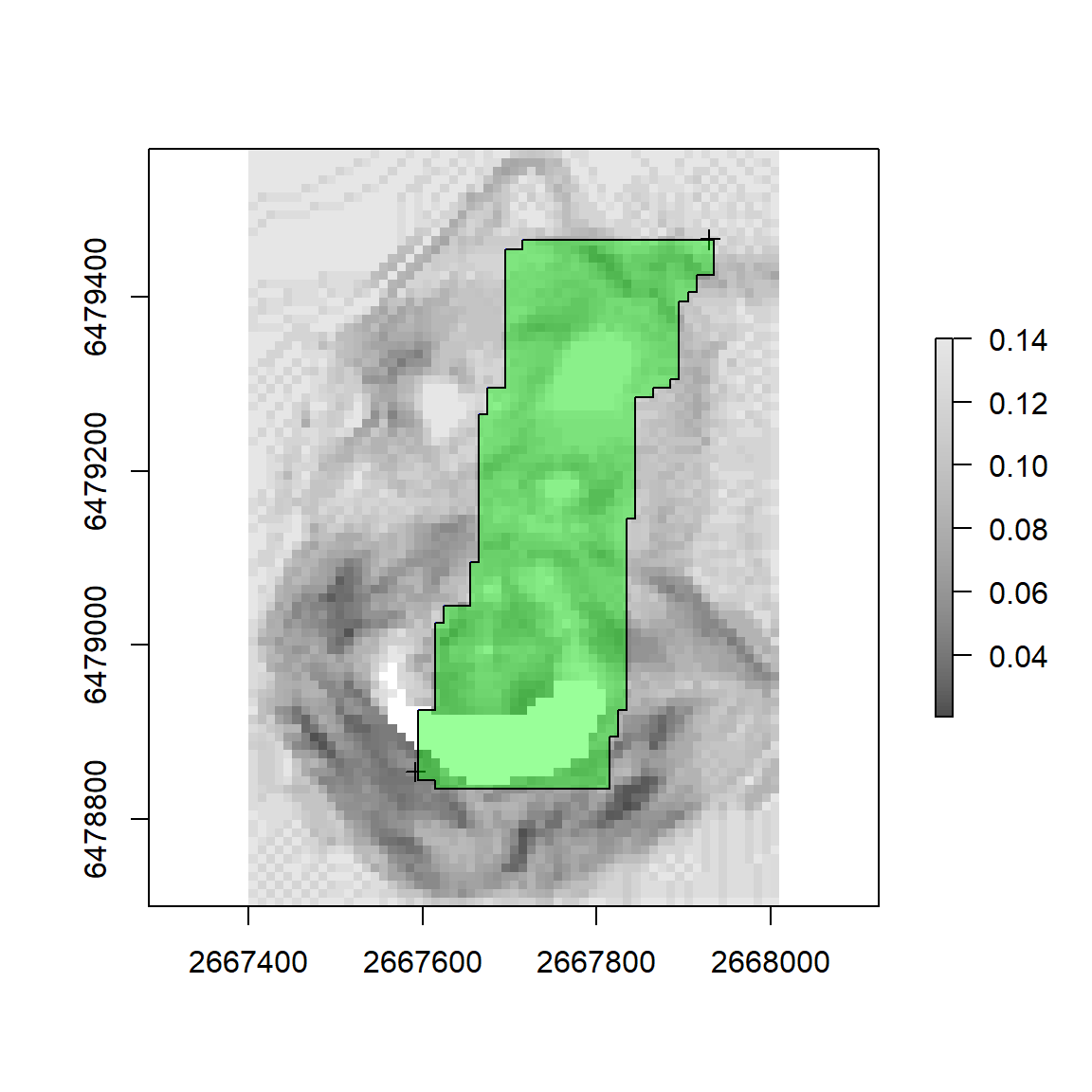## 2.5 Calculating Cost Corridors

# calculates accumulated cost surface from A and from B and B to A. These two cost surfaces are averaged. If rescale argument TRUE (not default) then final accumulated cost surface rescaled to 1.

cc <- leastcostpath::create_cost_corridor(cost_surface = slope_altitude_cs, origin = A, destination = B, rescale = TRUE)

plot(cc, col = heat.colors(100))
plot(A, add = T, col = "white", pch = 16)
plot(B, add = T, col = "white", pch = 16)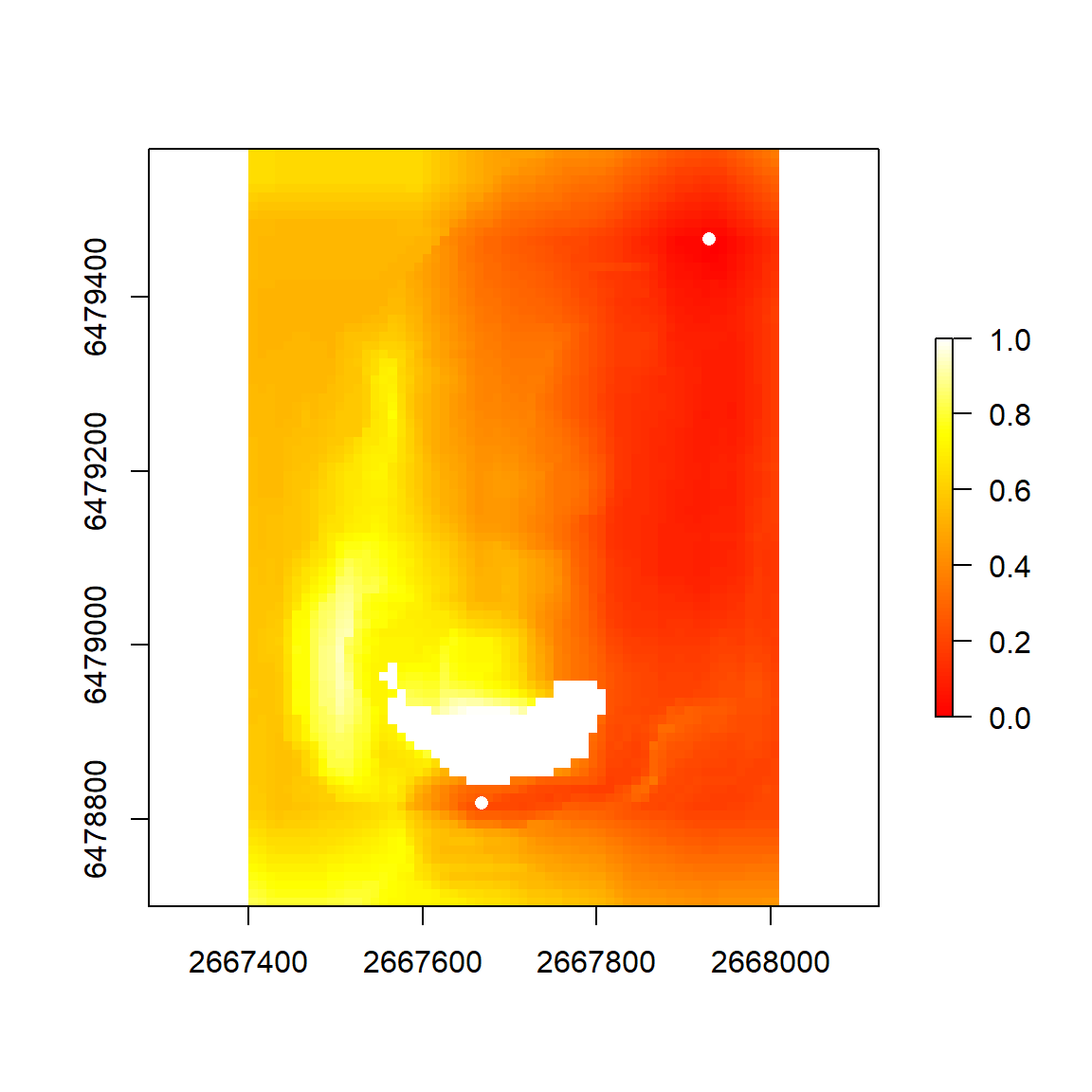## 2.6 Calculating From-Everywhere-To-Everywhere Least Cost Paths

set.seed(1)
random_locs <- sp::spsample(x = dem_extent, n = 4, type = 'regular')

# if parallel is TRUE then need to specify number of cores via ncores argument
fete_lcp <- leastcostpath::create_FETE_lcps(cost_surface = slope_cs, locations = random_locs, cost_distance = FALSE, parallel = FALSE)

plot(raster(slope_altitude_cs), col = grey.colors(100))
plot(fete_lcp, add = T, col = "red")
plot(random_locs, add = T, col = "black", pch = 16)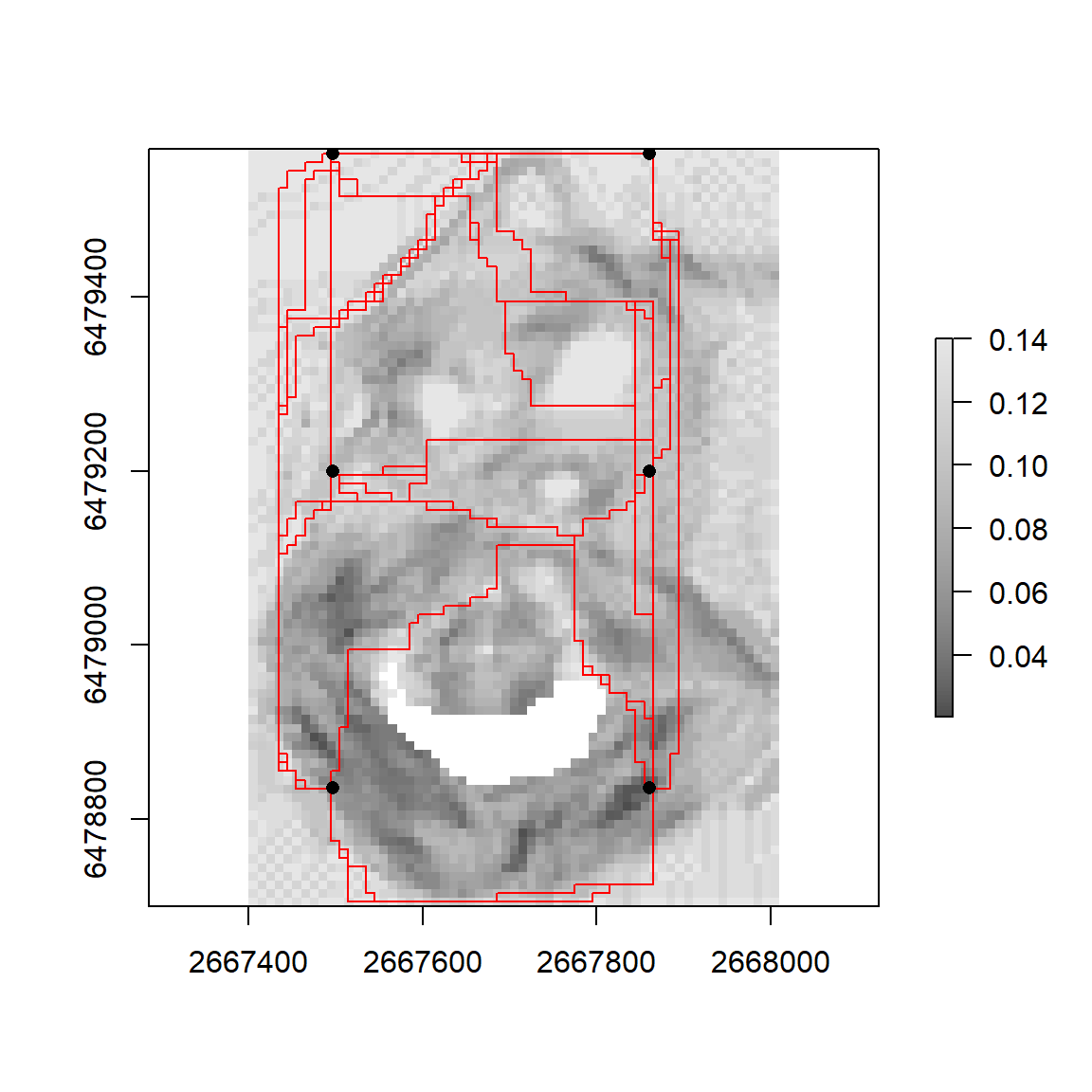## 2.7 Least Cost Path Density

# rasterises the LCPs and calculates the number of times an LCP crosses a raster cell. If rescale argument TRUE (not default) then LCP density rescaled to 1. If rasterize_as_points argument TRUE (default) then LCP vertices points are rasterized. This is quicker than rasterizing lines (rasterize_as_lines argument FALSE) but will contain 'gaps' where no LCP vertex point is present
fete_lcp_density <- leastcostpath::create_lcp_density(lcps = fete_lcp, raster = dem, rescale = TRUE, rasterize_as_points = TRUE)

fete_lcp_density[fete_lcp_density == 0] <- NA

plot(fete_lcp_density, col = heat.colors(100))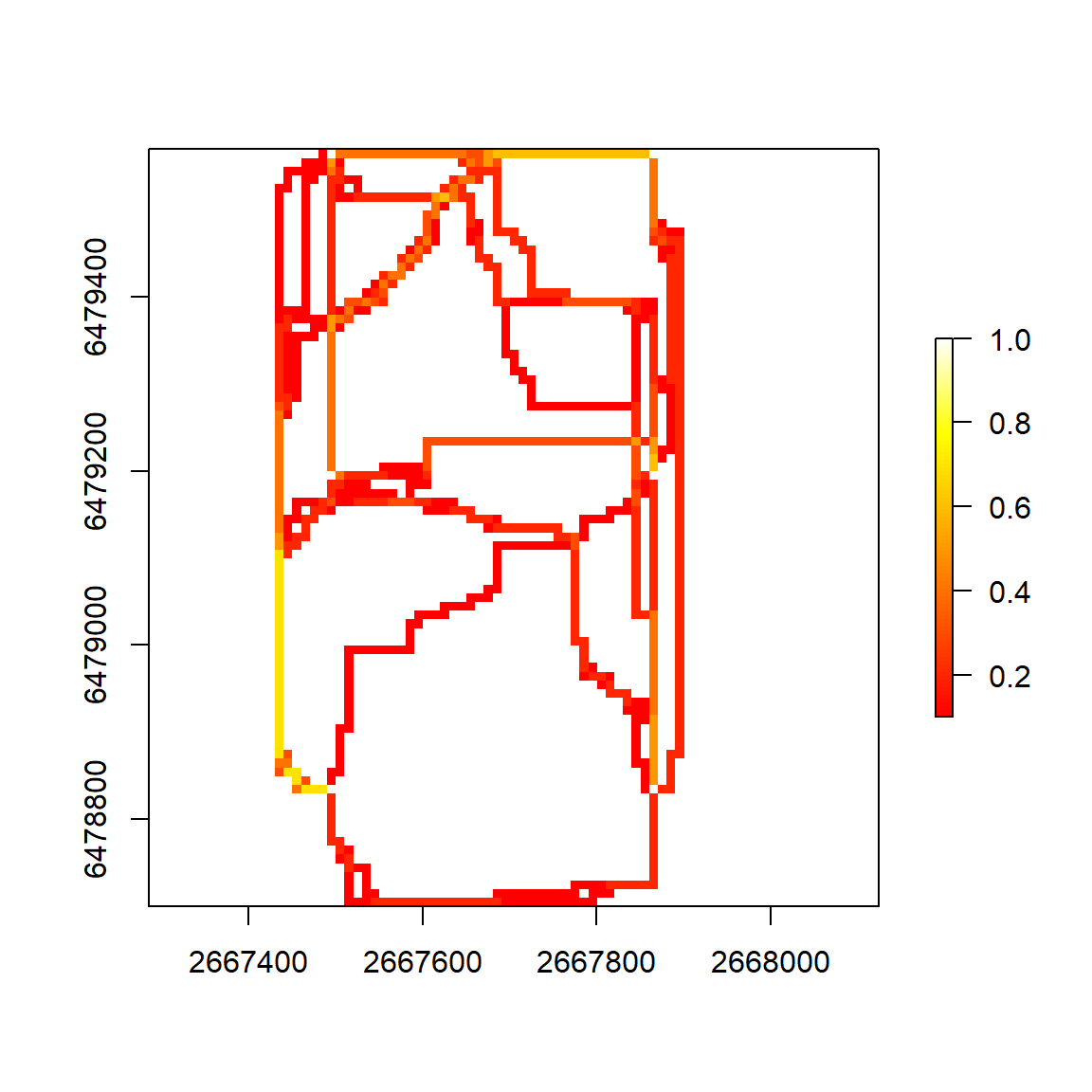# reset set.seed to NULL
set.seed(NULL)